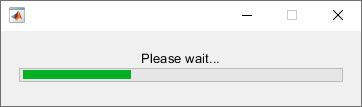# send

Send data from worker to client using a data queue

## Syntax

``send(queue, data)``
``send(pollablequeue, data)``

## Description

example

````send(queue, data)` sends a message or data with the value `data` to the `parallel.pool.DataQueue` specified by `queue`. Call `afterEach` to pass each of the pending messages to the function specified by `afterEach`. ```

example

````send(pollablequeue, data)` sends a message or data with the value `data` to the `parallel.pool.PollableDataQueue` specified by `pollablequeue`. Retrieve the result using `poll``(pollablequeue)`, and return `data` as the answer.Use the `send` and `poll` functions together using a pollable data queue to transfer and retrieve messages or data from different workers.```

## Examples

collapse all

Construct a `DataQueue`, and call `afterEach`.

```q = parallel.pool.DataQueue; afterEach(q, @disp); ```
Start a `parfor`-loop, and send a message. The pending message is passed to the `afterEach` function, in this example `@disp`.

```parfor i = 1:3 send(q, i); end;```
``` 1 2 3```

For more details on listening for data using a `DataQueue`, see `afterEach`.

Construct a `PollableDataQueue`.

```p = parallel.pool.PollableDataQueue; ```
Start a `parfor`-loop, and send a message, such as data with the value 1.
```parfor i = 1 send(p, i); end ```
Poll for the result.

`poll(p)`
``` 1 ```

For more details on retrieving data using a `PollableDataQueue`, see `poll`.

This example shows a function that creates a `parfor` wait bar. Create a `DataQueue`, and use `afterEach` to specify the function to execute each time the queue receives data. This example calls a subfunction that updates the wait bar.

Create a `parfor`-loop to carry out a computationally demanding task in MATLAB®. Use `send` to send some dummy data on each iteration of the `parfor`-loop. When the queue receives the data, `afterEach` calls `nUpdateWaitbar` in the client MATLAB, and you can observe the wait bar progress.

```function a = parforWaitbar D = parallel.pool.DataQueue; h = waitbar(0, 'Please wait ...'); afterEach(D, @nUpdateWaitbar); N = 200; p = 1; parfor i = 1:N a(i) = max(abs(eig(rand(400)))); send(D, i); end function nUpdateWaitbar(~) waitbar(p/N, h); p = p + 1; end end ```## Input Arguments

collapse all

Data queue, specified as a `parallel.pool.DataQueue` object.

Example: `q = parallel.pool.DataQueue;`

Message or data from workers to a data queue, specified as any data type that can be serialized.

Example: `send(queue, data);`

Pollable data queue, specified as a `parallel.pool.PollableDataQueue` object.

Example: ```p = parallel.pool.PollableDataQueue;```